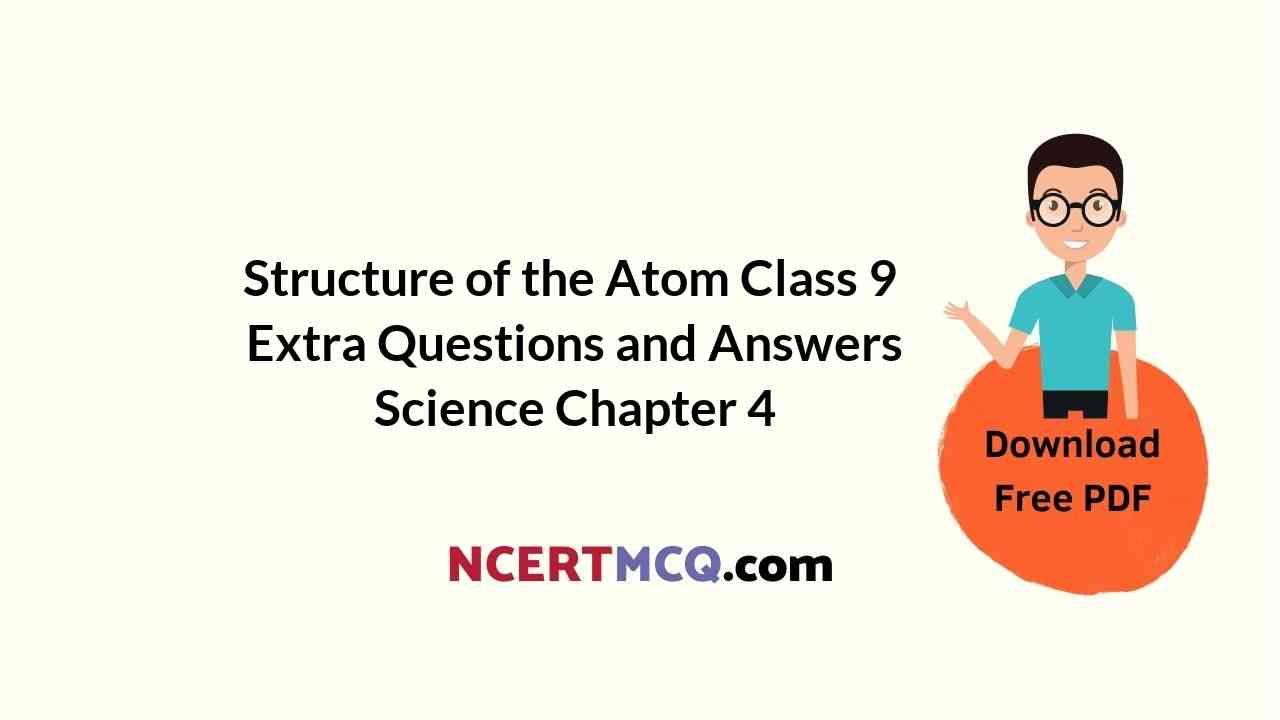In this page, we are providing Structure of the Atom Class 9 Extra Questions and Answers Science Chapter 4 pdf download. NCERT Extra Questions for Class 9 Science Chapter 4 Structure of the Atom with Answers will help to score more marks in your CBSE Board Exams. https://ncertmcq.com/extra-questions-for-class-9-science/

## Class 9 Science Chapter 4 Extra Questions and Answers Structure of the Atom

Extra Questions for Class 9 Science Chapter 4 Structure of the Atom with Answers Solutions

### Structure of the Atom Class 9 Extra Questions Very Short Answer Type

Structure Of Atom Class 9 Extra Questions Question 1.
Is it possible for the atom of an element to have one electron, one proton and no neutron? If so, name the element. [NCERT Exemplar]
Yes, it is true for hydrogen atom which is represented as $$_{ 1 }^{ 1 }{ H }$$

Class 9 Science Chapter 4 Extra Questions Question 2.
Will 35Cl and 35Cl have different valencies? Justify your answer. [NCERT Exemplar]
No, 35Cl and 35Cl are isotopes of an element.

Class 9 Structure Of Atom Extra Questions Question 3.
Why did Rutherford select a gold foil in his a-ray scattering experiment? [NCERT Exemplar]
Rutherford selected a gold foil in his a-ray scattering experiment because gold has high malleability.

Structure Of Atom Class 9 Important Questions With Answers Pdf Question 4.
One electron is present in the outermost shell of the atom of an element X. What would be the nature and value of charge on the ion formed if this electron is removed from the outermost shell? [NCERT Exemplar]
+ 1.

Extra Questions On Structure Of Atom Class 9 Question 5.
Write any two observations which support the fact that atoms are divisible. [NCERT Exemplar]
Discovery of electrons and protons.

Structure Of Atom Extra Questions Question 6.
Write down the electron distribution of chlorine atom. How many electrons are there in a L-shell? (Atomic number of chlorine is 17). [NCERT Exemplar]
The electron distribution of chlorine atom is 2, 8, 7 and the L-shell has 8 electrons.

Class 9 Science Chapter 4 Extra Questions And Answers Question 7.
In the atom of an element X, 6 electrons are present in the outermost shell. If it acquires noble gas configuration by accepting requisite number of electrons, then what would be the charge on the ion so formed? [NCERT Exemplar]
– 2.

Extra Questions Of Structure Of Atom Class 9 Question 8.
Helium atom has 2 electrons in its valence shell but its valency is not 2, Explain.
Helium atom has 2 electrons in its outermost shell and its duplet is complete. Hence the valency is zero.

Structure Of Atom Extra Questions Class 9 Question 9.
An element X has a mass number 4 and atomic number 2. Write the valency of this element.
Valency is zero, as K-shell is completely filled.

Class 9 Science Ch 4 Extra Questions Question 10.
Who identified the sub-atomic particle electron?
J.J. Thomson.

Class 9 Chapter 4 Science Extra Questions Question 11.
Name the rays discovered by E. Goldstein.
Canal rays.

Structure Of An Atom Class 9 Extra Questions Question 12.
Give two examples from everyday life where we use cathode ray tubes.
Television picture tube and Fluorescent light tubes.

Structure Of Atom Class 9 Extra Questions And Answers Question 13.
What are the actual values of charge and mass of the electron?
Charge = 1.60 x 10-19 C (one unit negative charge)
Mass = 9.11 x 10-31 kg (1/1840th of the mass of hydrogen atom).

Chapter 4 Science Class 9 Extra Questions Question 14.
Compare the radius of the nucleus with that of the atom.
Radius of the atom is of the order of 10-10 m while that of the nucleus is of the order of 10-15 m. Thus, nucleus is 10-15/10-10 i.e., 1/100,000th of the size of the atom.

Ch 4 Science Class 9 Extra Questions Question 15.
Why are Bohr’s orbits called stationary states?
According to Bohr, the orbits in which the electrons revolve have fixed energies. Hence, they are called stationary states (stationary means fixed).

Question 16.
Who discovered neutron?

Question 17.
What are nucleons? What is their number called?
Protons and neutrons present in the nucleus are collectively called nucleons. Their total number is called ‘mass number’.

Question 18.
What do you mean by ‘Atomic number’?
Atomic number of an element is the number of protons present in the nucleus of the atom of that element.

Question 19.
Who discovered the nucleus of the atom?
Rutherford.

Question 20.
What is the charge on alpha particle?
+ 2.

Question 21.
The mass number of an element is 18. It contains 7 electrons. What is the number of protons and neutrons in it?
Number of protons = 7 Number of neutrons = 11 ,

Question 22.
What is the maximum number of electrons that can be present in M-shell?
M-shell means 3rd shell for which n = 3. Hence, maximum number of electrons that can be present in M-shell = 2n2 = 2 x 32 = 18.

Question 23.
Identify the isotopes out of A, B, C and D? 33A17, 40B20, 37C17, 38D19.
33A14 and 37C17? are isotopes.

Question 24.
Represent the three isotopes of hydrogen and give their names.
$$_{ 1 }^{ 1 }{ H }$$ (Protium), $$_{ 2 }^{ 1 }{ H }$$ (Deuterium), $$_{ 3 }^{ 1 }{ H }$$ (Tritium).

Question 25.
How many electrons are present in the species He2+ ion? Suggest another name for it.
He2+ ion has no electrons. It is also called alpha (α) particle.

Question 26.
What is the reason for the identical chemical properties of all the isotopes of an element?
Isotopes have identical electronic configuration.

Question 27.
Give one similarity and one difference between a pair of isotopes.
They have same number of protons but different number of neutrons in their nuclei.

Question 28.
Give the number of protons and neutrons in an atom of uranium (U-235) used in a nuclear reactor.
In nuclear reactor U-235 is used. Atomic number of Uranium is 92.
∴ Number of protons = Atomic number = 92
Number of neutrons = Mass number – Atomic number = 235 – 92 = 143.

Question 29.
Out of proton and neutron, which is heavier?
Neutron is slightly heavier (1.675 x 10-27 kg) than proton (1.67 x 10-27 kg).

Question 30.
If K and L shells of an atom are completely filled what will be its name?
The atom will belong to the noble gas element neon (Ne).

Question 31.
Do isobars have also identical chemical characteristic like isotopes?
No, these are not identical because the isobars have different atomic number as well as different electronic configuration.

Question 32.
What is the number of electrons in the valence shell of chlorine (Z = 17)?
The electronic distribution of the element is:
K(2), L(8), M(7). This means that the valence shell of chlorine has 7 electrons.

Question 33.
Which radioisotope is used for the treatment of cancer?
Radioisotope Co-60 is used for the treatment of cancer.

Question 34.
Out of C-12 and C-14 isotopes of carbon, which is of radioactive nature?
C-14 isotope is of radioactive nature.

Question 35.
The electron configuration of an element is: 2(K), 8(L), 5(M). Predict its valency.
The valency of the element is 3. It is calculated as: 8 – 5 = 3.

Question 36.
Will $$_{ 12 }^{ 6 }{ C }$$ and $$_{ 14 }^{ 6 }{ C }$$ have different valencies?
Both the species are the isotopes of the same element i.e., the carbon. Since their atomic numbers Eire same, their electronic configurations as well as valencies will also be the same.

Question 37.
Do the elements $$_{ 3 }^{ 1 }{ X }$$ and $$_{ 3 }^{ 2 }{ Y }$$ represent pair of isotopes?
No, they do not because the two elements differ in their atomic numbers. Isotopes have the same atomic numbers.

### Structure of the Atom Class 9 Extra Questions Short Answer Type 1

Question 1.
In response to a question, a student stated that in an atom, the number of protons is greater than the number of neutrons, which in turn is greater than the number of electrons. Do you agree with the statement? Justify your answer. [NCERT Exemplar]
No, the statement is incorrect. In an atom the number of protons and electrons is always equal.

Question 2.
Calculate the number of neutrons present in the nucleus of an element X which is represented as $$_{ 31 }^{ 15 }{ X }$$. [NCERT Exemplar]
Mass number = No. of protons + No. of neutrons = 31
∴ Number of neutrons = 31 – number of protons
= 31 – 15
= 16

Question 3.
The atomic number of calcium and argon are 20 and 18 respectively, but the mass number of both these elements is 40. What is the name given to such a pair of elements? [NCERT Exemplar]
∴ They are isobars because isobars have different atomic numbers but same mass number.

Question 4.
Why do Helium, Neon and Argon have a zero valency?
Helium has two electrons in its only energy shell, while Argon and Neon have 8 electrons in their valence shells. As these have maximum number of electrons in their valence shells, they do not have any tendency to combine with other elements. Hence, they have a valency equal to zero.

Question 5.
What are isobars? Give one example.
Isobars are the atoms of different elements which have different atomic numbers but same mass number.
Example is $$_{ 40 }^{ 18 }{ Ar }$$, $$_{ 40 }^{ 20 }{ Ca }$$ .

Question 6.
What is the number of valence electron in:
(i) Sodium ion (Na+)
(ii) Oxide ion (O2-)?
(i) Sodium ion (Na+)
No. of electrons: (11 – 1); Electronic configuration = 2, 8.
∴ Na+ ion has 8 valence electrons.

(ii) Oxide ion (O2-)
No. of electrons: (8 + 2) = 10; Electronic configuration = 2, 8.
∴ O2- ion has 8 valence electrons.

Question 7.
The number of electrons in the outermost ‘L’ shell of an atom is 5.
(a) Write its electronic configuration.
(b) What is its valency and why?
(a) 2, 5
(b) 3, because it needs three more electrons to complete its octet.

Question 8.
Write two difference between isobars and isotopes.
Isobars

• Same mass number but different atomic numbers.
• Different number of protons.

Isotopes:

• Same atomic number but different mass numbers.
• Same number of protons.

Question 9.
Mention the postulates Neils Bohr put forth to overcome the objections raised against Rutherford’s atomic model.
Bohr put forward the following postulates in order to overcome the objections raised against Rutherford s atomic method: .

Only certain special orbits known as discrete orbits of electrons are allowed inside the atom.
While revolving in discrete orbits the electrons do not radiate energy. These orbits or shells are called energy levels.

Question 10.
Given that natural sample of iron has isotopes $$_{ 54 }^{ 28 }{ Fe }$$, $$_{ 58 }^{ 28 }{ Fe }$$ and $$_{ 57 }^{ 28 }{ Fe }$$ in the ratio of 5%, 90% and 5% respectively. What will be the average atomic mass of iron (Fe)?
Average atomic mass
= $$\frac { 54 × 5 + 56 × 90 + 57 × 5 }{ 100 }$$
= $$\frac { 270+5040+285 }{ 100 }$$
= 55.95 u.

Question 11.
(a) What are canal rays? Who discovered them?
What is the charge and mass of canal ray?
(b) How are the canal rays different from electron in terms of charge and mass?
(a) New radiations in a gas discharge tube which are positively charged. E. Goldstein discovered them. Charge on canal rays is positive and mass is one unit.

(b) Electrons are negatively charged and their mass is approximately 1/2000 of that of canal rays.

Question 12.
The average atomic mass of a sample of an element y is 35.5 u. What are the percentages of isotopes $$_{ 37 }^{ 17 }{ y }$$ and $$_{ 35 }^{ 17 }{ y }$$, in the sample?
Let the % composition of $$_{ 37 }^{ 17 }{ y }$$ be x.
Then the % composition of $$_{ 37 }^{ 17 }{ y }$$ will be 100 – x
Now,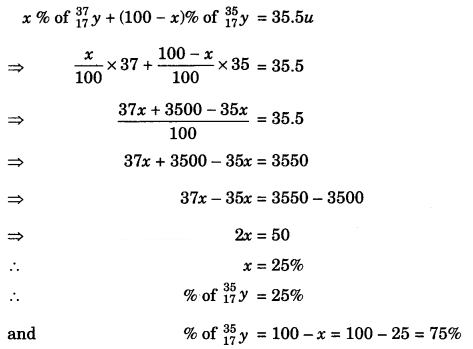Question 13.
(i) Write the postulates of Bohr model of atom.
(ii) Draw a sketch of Bohr model of an atom with atomic number 15.
(i) Only certain special orbits-discrete orbits of electrons are allowed.

(ii) While revolving in discrete orbits, the electrons do not radiate energy.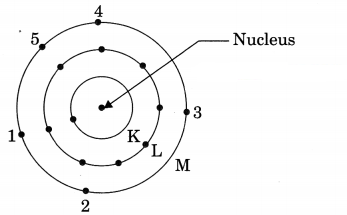Question 14.
List the main differences between an atom and an ion.
Atom:

• It is electrically natural.
• The valence shell of atom may or may not have 8 electrons.
• The atoms are less stable.
• It may or may not exist freely in solution.

Ion:

• It has positive or negative charge.
• The valence shell of an ion has 8 electrons.
• The ions are more stable.
• It can exist freely in solution.

Question 15.
An ion has one neutron more than the number of protons but has one electron less than the number of protons. Which two ions are possible which satisfy this condition? Explain.
(i) Na+ ion, because it has atomic number = 11 and mass number = 23. Thus, no. of protons = 11, no. of neutrons = 23 – 11 = 12 and number of electrons = 11 – 1 = 10.

(ii) K+ ion, because it has atomic number = 19 and mass number = 39. Thus, no. of protons = 19, No. of neutrons = 39 – 19 = 20 and no. of electrons = 19 – 1 = 18.

Question 16.
Give the main points of Dalton’s atomic theory.

• All matter is made up of a large number of extremely small indivisible particles called atoms.
• Atoms of the different elements are different.
• Atom is the smallest unit of matter which takes part in a chemical reaction.
• Atoms can neither be created nor be destroyed.

Question 17.
The number of electrons, protons and neutrons in a species are equal to 18, 16 and 16 respectively. Assign proper symbol of the species.
Number of electrons present = 18
Number of neutrons present = 16
Atomic number of the element = 16
The element with atomic number 16 is sulphur (S). Since it has 18 electrons and not 16, it is therefore, an anion (S2- ion).

Question 18.
An element with mass number 81 contains 31.7% more neutrons as compared to protons. Assign the atomic symbol. [NCERT Exemplar]
We know
Mass number = No. of protons + No. of neutrons = 81
i.e., p + n = 81
Let number of protons = x### Structure of the Atom Class 9 Extra Questions Short Answer Type 2

Question 1.
Show diagrammatically the electron distributions in a sodium atom and a sodium ion and also given their atomic number. [NCERT Exemplar]Since the atomic number of sodium atom is 11, it has 11 electrons. A positively charged sodium ion (Na+) is formed by the removal of one electron from a sodium atom. So, a sodium ion has 11 – 1 = 10 electrons. Thus, electronic distribution of sodium ion will be 2, 8. The atomic number of an element is equal to the number of protons in its atom. Since, sodium atom and sodium ion contain the same number of protons, therefore, the atomic number of both is 11.

Question 2.
The ratio of the radii of hydrogen atom and its nucleus is ~ 105. Assuming the atom and the nucleus to be spherical. [NCERT Exemplar]
(i) What will be the ratio of their sizes?
(ii) If atom is represented by planet Earth ‘Re’ = 6.4 x 105 m, estimate the size of the nucleus.
(i) Volume of the sphere = $$\frac { 4 }{ 3 }$$ πr3
Let R be the radius of the atom and r be that of the nucleus.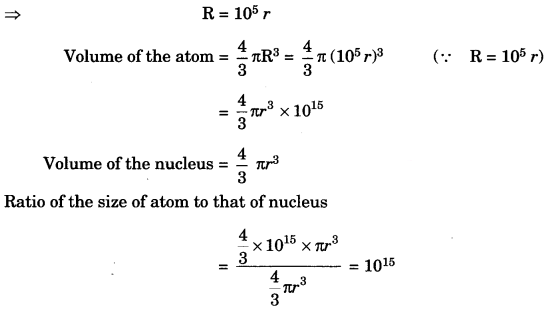(ii) If the atom is represented by the planet earth (Re = 6.4 x 106 m) then the radius of the nucleus
would be rn = $$\frac{\mathrm{R}_{e}}{10^{5}}$$
rn = $$\frac{6.4 \times 10^{6} \mathrm{m}}{10^{5}}$$
= 6.4 x 10 m = 64 m.

Question 3.
The number of protons, neutrons and electrons in particles from A to E are given below: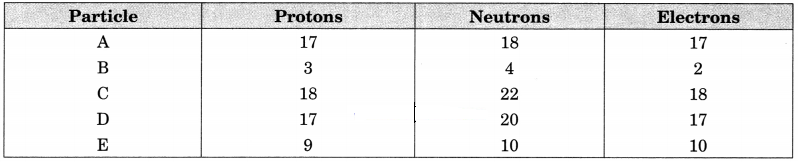(i) Which one is a cation?
(ii) Which one is an anion?
(iii) Which represent pair of isotopes?
(i) B is a monovalent cation (B+)
(ii) E is a monovalent anion (E)
(iii) A and D represent pair of isotopes.

Question 4.
Explain Bohr Bury rules for distribution of electrons into different shells. Write the distribution of electrons in sodium atom (Z = 11).
Bohr Bury Rules:

• The maximum no. of electrons present in a shell is given by the formula 2n2 (where n is shell number).
• The maximum number of electrons that can be accommodated in the outermost orbit is 8.
• Electrons are not accommodated in a given shell, unless the inner shells are filled.
• Electronic configuration of Na = 2, 8, 1.

Question 5.
Atom A has a mass number 238 and atomic number 92 and atom B has mass number 235 and atomic number 92.
(i) How many protons, atoms A and B have?
(ii) How many neutrons, atoms A and B have?
(iii) Are atoms A and B isotopes of the same element? How?
(i) Protons in atoms A and B = 92
(ii) Neutrons in atoms A and B = 146 and 143
(iii) Yes, atom A and B are isotopes of the element since they have the same atomic number or same electronic configuration.

Question 6.
State three features of the nuclear model of an atom put forward by Rutherford.

1. There is a positively charged centre in an atom called the nucleus. Nearly, all the mass of an atom resides in the nucleus.
2. The electrons revolve around the nucleus in well-defined orbits.
3. The size of the nucleus is very small as compared to the size of the atom.

Question 7.
The nucleus of an atom is found to have a total mass of nearly 20.088 x 10-27 kg and a total charge of 9.612 x 10-19 coulombs. Calculate the atomic number and mass number of the atom. Name the element.
Mass of a nucleon (proton or neutron)
= 1.674 x 10-27 kg
Total mass of nucleus = 20.088 x 10-27 kg
∴ No. of nucleons (protons + neutrons) present in the nucleus
= $$\frac{20.088 \times 10^{-27}}{1.674 \times 10^{-27}}$$ = 12
∴ Mass number = 12u.
Charge on one proton = 1.602 x 10-19 coulombs; Total charge on the nuclear = 9.612 x 10-19
∴ No.of protons in the nucleus
= $$\frac{9.612 \times 10^{-19}}{1.602 \times 10^{-19}}$$ = 6
Atomic number = 6. Thus, the element is carbon.

Question 8.
Give reasons why?
(а) Atom is electrically neutral.
(b) Atom as a whole is an empty space.
(c) Rutherford model of atom could not provide stability to the nucleus.
(a) An atom is electrically neutral because the number of protons and number of electrons in it are equal.

(b) According to Rutherford’s experiment, the size of the nucleus is very small as compared to the size of an atom, therefore, atom as a whole is an empty space.

(c) According to Rutherford, the protons are present inside the nucleus and electrons revolve around the nucleus. According to the elecromagnetic theory, a charged particle moving in a circular path continuously loses energy in the form of electromagnetic radiations and finally falls into the nucleus.

Question 9.
Write the complete symbol for
(i) the nucleus with atomic number 56 and mass number 138.
(ii) the nucleus with atomic number 26 and mass number 55.
(iii) the nucleus with atomic number 4 and mass number 9.
(i) The element with atomic number 56 is Ba. Its symbol is $$_{ 138 }^{ 56 }{ Ba }$$

(ii) The element with atomic number 26 is Fe. Its symbol is $$_{ 56 }^{ 26 }{ Fe }$$

(iii) The element with atomic number 4 is Be. Its symbol for $$_{ 9 }^{ 4 }{ Be }$$

Question 10.
How many protons, electrons and neutrons are there in the following nuclei?
(i) $$_{ 17 }^{ 8 }{ O }$$
(ii) $$_{ 25 }^{ 12 }{ Mg }$$
(iii) $$_{ 80 }^{ 35 }{ Br }$$
(i) $$_{ 35 }^{ 79 }{ Br }$$
Atomic number, Z = 8
Mass number, A = 17
No. of protons = No. of electrons = Z = 8
No. of neutrons + No. of protons = A
No. of neutrons + 8 = 17
or No. of neutrons = 17 – 8 = 9

(ii) $$_{ 25 }^{ 12 }{ Mg }$$
Atomic number, Z = 12
Mass number, A = 25
No. of protons = No. of electrons = Z = 12
No. of neutrons = A – No. of protons = 25 – 12 = 13.

(iii) $$_{ 80 }^{ 35 }{ Br }$$
Atomic number, Z = 35
Mass number, A = 80
No. of protons = No. of electrons = Z = 35
No. of neutrons = A – No. of protons = 80 – 35 = 45.

Question 11.
An ion with mass number 56 contains 3 units of positive charge and 30.4% more neutrons than electrons. Assign the symbol for the ion.
Since the iron carries 3 units of positive charge, it will have 3 electrons less than the number of protons. Let number of electrons = x
No. of protons = x + 3
No. of neutrons = x + $$\frac { x × 30.4 }{ 100 }$$
= x + 0.304x
= 1.304x
Now, No. of protons + No. of neutrons = 56
x + 3 + 1.304 x = 56
2.304 x = 53
x = $$\frac { 53 }{ 2.304 }$$ = 23
No. of electrons = 23
No. of protons = 23 + 3 = 26
Symbol = $$_{ 56 }^{ 26 }{ Fe }$$3+.

Question 12.
The nuclear radius is of the order of 10-13 cm while atomic radius is of the order 10-8 cm. Assuming the nucleus and the atom to be spherical, what fraction of the atomic volume is occupied by the nucleus?
The volume of a sphere = 4 πR3/3 where R is the radius of the sphere.
∴ Volume of the nucleus = 4 πr3/3 = 4π (10-13)3/3 cm3
Similarly,
Volume of the atom = 4 πR3/3 = 4π (10-8)3/3 cm3
∴ Fraction of the volume of atom occupied by the nucleus
= $$\frac{4 \pi\left(10^{-13}\right)^{3} / 3 \mathrm{cm}^{3}}{4 \pi\left(10^{-8}\right)^{3} / 3 \mathrm{cm}^{3}}$$
= 10-15.

Question 13.
Calculate the number of electrons, protons and neutrons in the following species:
(i) Phosphorus atom
(ii) Phosphide ion (P3-)
(iii) Magnesium ion (Mg2+)
Mass numbers: P = 31; Mg = 24
Atomic numbers: P = 15, Mg = 12.
(i) Phosphorus atom
Number of electrons = Atomic number = 15
Number of protons = Atomic number = 15
Number of neutrons = Mass number – Atomic number
= 31 – 15 = 16.

(ii) Phosphide ion (P3-)
Phosphide ion (P3-)
= Phosphorus atom + 3 electrons
P3- = P + 3e
Thus, phosphide ion has same number of protons and neutrons as phosphorus atom but has three electrons more.
Number of electrons = 15 + 3 = 18
Number of protons = 15
Number of neutrons = 31 – 15 = 16

(iii) Magnesium ion (Mg2+)
Mg2+ ion is formed by loss of two electrons by Mg atom. Therefore, it has two electrons less than number of electrons in Mg atom.
Mg2+ = Mg – 2e
Number of electrons = 12 – 2 = 10
Number of protons = 12
Number of neutrons = (24 – 12) = 12

### Structure of the Atom Class 9 Extra Questions Long Answer Type

Question 1.
(a) Enlist the conclusions drawn by Rutherford from his a-ray scattering experiment.
(b) In what way is the Rutherford’s atomic model different from that of Thomson’s atomic model?
(a) Rutherford concluded from the a-particle scattering experiment that:

(i) Most of the space inside the atom is empty because the a-particles passed through the gold foil without getting deflected.

(ii) Very few particles were deflected from their path, indicating that the positive charge of the atom occupies very little space.

(iii) A very small fraction of a-particles were deflected by 180°, indicating that all the positive charges and mass of the gold atom were concentrated in a very small volume within the atom.

From the data he also calculated that the radius of the nucleus is about 105 times less than the radius of the atom.

(b) Rutherford proposed a model in which electrons revolve around the nucleus in well-defined orbits. There is a positively charged center in an atom called the nucleus. He also proposed that the size of the nucleus is very small as compared to the size of the atom and nearly all the mass of an atom is centred in the nucleus.

Whereas, Thomson proposed the model of an atom to be similar to a Christmas pudding. The electrons are studded like currants in a positively charged sphere like Christmas pudding and the mass of the atom was supposed to be uniformly distributed.

Question 2.
(a) What were the drawbacks of Rutherford’s model of an atom?
(b) What are the postulates of Bohr’s model of an atom?
(a) The orbital revolution of the electron is not excepted to be stable. Any particle in a circular orbit would undergo an acceleration and the charged particles would radiate energy. Thus, the revolving electron would lose energy and finally fall into the nucleus. If this were so, the atom should be highly unstable and hence matter would not exist in the form that we know.

(b) The postulates put forth by Neils Bohr’s about the model of an atom:
(i) Only certain special orbits known as discrete orbits of electrons, are allowed inside the atom.

(ii) While revolving in discrete orbits the electrons do not radiate energy.
These orbits are called energy levels. Energy levels in Em atom are shown by circles.
These orbits are represented by the letters K, L, M, N, …………….. or the numbers, n = 1, 2, 3, 4, ………………

Question 3.
An atom of an element has three electrons in the third shell which is the outermost shell. Write
(a) the electronic configuration
(b) the atomic number
(c) number of protons
(d) valency
(e) the name of the element
(f) its nature whether metal or non-metal.
The third shell is M-shell. If the atom of the element has three electrons in the third shell, this means that K and L shells are already filled.
(a) Electronic configuration: 2, 8, 3
(b) Atomic number = No. of electrons = 13
(c) Number of protons = No. of electrons = 13
(d) Valency of the element = 3
(e) The element with Z = 13 is aluminium (Al)
(f) It is a metal.

Question 4.
Answer the following in one line or two:
(a) What is the maximum number of electrons that can be accommodated in the outermost energy shell in an atom?
(b) On the basis of Thomson’s model of an atom, explain how an atom is neutral as a whole.
(c) How many neutrons are present in hydrogen atom?
(d) Do isobars belong to the same element?
(e) An element has five electrons in the M shell which is the outermost shell. Write its electronic configuration.
(a) The outermost energy shell in an atom can have a maximum of eight electrons.

(b) According to Thomson’s model of an atom, all the protons in an atom are present in the positively charged sphere while negatively charged electrons are studded in this sphere. Since the electrons and protons are equal in number each carrying one unit charge, the atom as a whole is electrically neutral.

(c) Hydrogen atom has no neutron.

(d) No, isolars belong to different elements since they differ in their atomic numbers.

(e) The electronic configuration of the element is K(2), L(8), M(5).

Question 5.
Explain why did Rutherford select a gold foil in his alpha particle scattering experiments? What observations in a-scattering experiment led Rutherford to make the following observations:
(i) Most of the space in an atom is empty.
(ii) Nucleus is positively charged.
Mention any two drawbacks of Rutherford’s model.
Rutherford selected a gold foil because he wanted a very thin layer as possible. This gold foil was about 1000 atoms thick.

(i) As most of the alpha particles passed through the foil undeviated, it means that they did not come across any obstruction in their part. Thus, most of the space in an atom was thought to be empty.

(ii) Very few particles deviated by small angles from their path which suggested that nucleus is positively charged.

The revolution of the electron in any circular orbit is not expected to be stable. Any particle in a circular orbit would undergo acceleration. During acceleration, charged particles would radiate energy. Thus, the revolving electrons would lose energy and finally fall into the nucleus. If this were so, the atom should be highly unstable and matter would not exist in the form that we know. We know that atoms are quite stable.

Question 6.
(a) Why are anode rays called canal rays?
(6) Mention two postulates of J.J. Thomson’s model.
(c) Compare the properties of protons and electrons.
(a) The anode rays produced at the anode of the discharge tube are called canal rays because they pass through the holes of the cathode.

(b)

• Atom consists of positively charged sphere and electrons are embedded in it.
• The negative and positive charges are equal in magnitude.

(c) Protons:

• Positively charged.
• Mass of 1 proton is equal to mass of H atom.

Electrons:

• Negatively charged.
• Mass of electron is 1/1840 times that of a proton.

Question 7.
(a) What are isobars?

(b) Atomic number of an element Y is 17.
(i) Write its electronic configuration.
(ii) What is the number of valence electron in Y?
(iii) How many electrons are needed to complete the octet of Y?
(iv) Is it a metal or non-metal?

(c) The valency of Na is 1 and not 7. Give reason.
(a) Atoms of different elements with different atomic numbers which have same mass number are called isobars.

(b) (i) 2,8, 7
(ii) 7
(iii) 1
(iv) Non-metal

(c) Electronic configuration of Na is 2, 8,1. It can lose one electron to attain the electronic configuration of neon. Therefore, the valency of Na is 1. The valency of Na may be 7 when it gains 7 electrons in its valence shell, but gain ring 7 electrons is difficult. Therefore, the valency of Na is not 7.

Question 8.
An atom of an element has 2 electrons in the M-shell i.e., third shell. What will be the atomic number of this element? Name this element. Find the valency of this element. Also, find the number of neutrons in the atom of this element.
(i) Atomic number is 12(ii) Element is magnesium.
(iii) Valency is 2 (+2)
(iv) No. of neutrons = Atomic mass – No. of protons = 24 – 12 = 12.

### Structure of the Atom Class 9 Extra Questions HOTS

Question 1.
What information do you get from the figure given below about the atomic number, mass number and valency of atoms X, Y and Z? Give your answer in a tabular form. [NCERT Exemplar]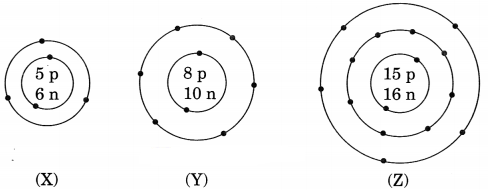Question 2.
In the Gold foil experiment of Geiger and Marsdein, that paved the way for Rutherford’s model of an atom, ~ 1.00% of the a-particles were found to deflect at angles > 50°. If one mole of α – particles were bombarded on the gold foil, compute the number of α – particles that would deflect at angles less than 50°. [NCERT Exemplar]
% of α – particles deflected more than 50° = 1% of α – particles
% of α – particles deflected less than 50° = 100 – 1 = 99%
Number of α – particles bombarded = 1 mole = 6.022 x 1023 particles
Number of particles that deflected at an angle less than 50°
= $$\frac { 99 }{ 100 }$$ x 6.022 x 1023
= $$\frac { 596.178 }{ 100 }$$ x 1023
= 5.96 x 1023.

Question 3.
An ion Y3- contains 18 electrons and 16 neutrons. Calculate the atomic number and mass number of the element Y. Name the element Y.
Number of electrons in Y3- ion = 18
Since negative charge is formed by gain of electrons by the neutral atom and the number of electrons gained is equal to the number of units of negative charge on the ion.
∴ Number of electrons in the neutral atom = 18-3 =15.
Now, for a neutral atom, Atomic number = Number of protons = Number of electrons.
∴ Atomic number of element Y = 15
Mass number of the element = Number of protons + Number of neutrons = 15 + 16 = 31
The given element Y with atomic number 15 is phosphorus.

Question 4.
The following data represent the distribution of electrons, protons and neutrons in atoms of four elements A, B, C, D.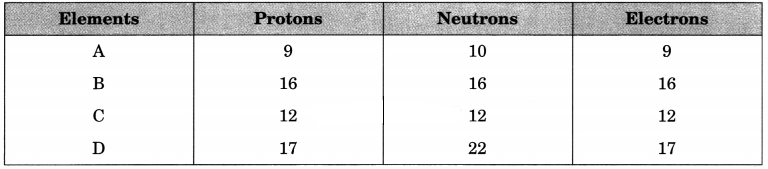(a) Give the electronic distribution of element B.
(b) The valency of element A.
(c) The atomic number of element B.
(d) The mass number of element D.
(а) Electronic distribution of elements B : 2, 8, 6.
(b) Valency of A is 1(-1).
(c) Atomic number of element B is 16.
(d) Mass number of element D is 39.

Question 5.
Give a reason to explain why:
(a) isotopes of an element show identical chemical properties?
(b) the atomic masses of elements are in fractions?
(c) atoms combine with other atoms?
(a) Since all the isotopes of an element have identical electronic configuration containing the same number of valence electrons. Therefore all the isotopes of an element show identical chemical properties.

(b) The fractional atomic masses of elements are due to their isotopes having different masses.

(c) The atoms combine with other atoms to achieve the electronic configuration of the nearest noble gas and thus, become more stable.

Question 6.
(a) Describe the main features of Bohr’s model of an atom. Draw a neat and labelled diagram of energy levels.
(b) Which of the following pairs are isotopes and which are isobars?
(i) $$_{ 58 }^{ 26 }{ A }$$, $$_{ 58 }^{ 28 }{ B }$$
(ii) $$_{ 79 }^{ 35 }{ X }$$, $$_{ 80 }^{ 35 }{ Y }$$
(c) Elements A and B have atomic numbers 18 and 16 respectively. Which of these two would be more reactive and why?
(a) Features of Bohr’s Models:
(i) Electrons revolve in certain permitted orbits which are associated with fixed amount of energy. So they are called energy levels (K, L, M, N) or sub-shells.
(ii) As long as electron revolves in the same energy level, they do not lose energy.
(iii) The energy of orbit closest to the nucleus is lowest and farthest away is highest.
(iv) When we supply energy to an electron, it goes to a higher energy level and when it comes back to a lower level it radiates energy.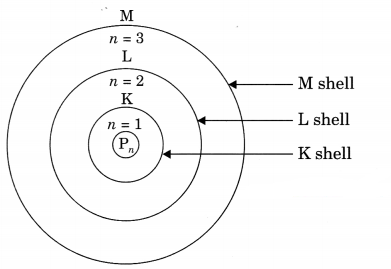(b) $$_{ 79 }^{ 35 }{ X }$$, $$_{ 80 }^{ 35 }{ Y }$$ are isotopes because their atomic no. is same but mass no. is different. $$_{ 58 }^{ 26 }{ A }$$, $$_{ 58 }^{ 28 }{ B }$$ are iso¬bars, as mass no. is same but atomic no. is different.

(c) B would be more reactive as its electronic configuration is 2, 8, 6 and it requires two more electrons to complete its octet.

Question 7.
Choose the noble gases from the elements shown in the table below: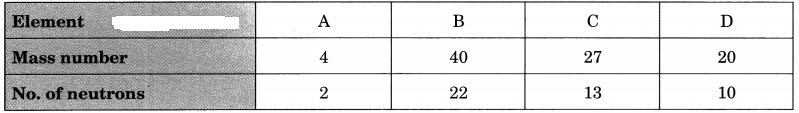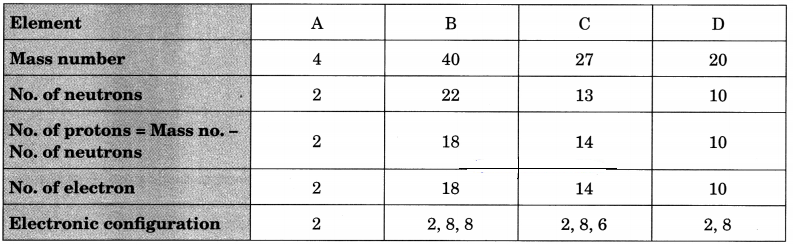A, B and D have completely filled valence shells, i.e., either 2 or 8 electrons in their valence shells.
∴ A, B and D are noble gases.

Question 8.
The mass number and electronic configuration of five elements A, B, C, D and E are as follows:(а) Name the elements which has 22 neutrons in nucleus.
(b) What is atomic number of C?
(c) Name the elements which will form most stable ionic bond.
(d) Give the formation of the compound between B and D.
(e) Name the elements which will not take part in chemical combination.
(a) E
(b) 11
(c) B and C
(d) DB4
(e) E

Question 9.
Write the electronic configurations of the following elements and write the number of valence electrons present in it.
(a) $$_{ 14 }^{ 7 }{ N }$$
(b) $$_{ 28 }^{ 14 }{ Si }$$
(c) $$_{ 40 }^{ 20 }{ Ca }$$
(d) $$_{ 40 }^{ 18 }{ Ar }$$
(e) $$_{ 9 }^{ 4 }{ Be }$$Question 10.
Complete the following table.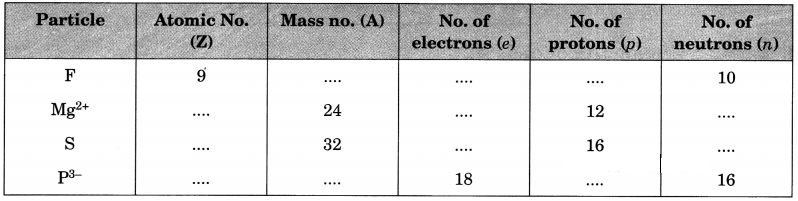For F atom
Atomic no. = 9 (Give)
No. of electrons = 9
No. of protons = 9
No. of neutrons = 10 (Given)
Mass no. = Z + n = 9 + 10 = 19

For Mg2+ ion
No. of protons = 12 (Given)
Atomic no. = 12
No. of electrons = 12 – 2 = 10
Mass no. = 24 (Give)
No. of neutrons = A – Z = 24 – 12 = 12

For S atom
No. of protons = 16 (Given)
Atomic no. = 16
No. of protons = 16
Mass no. = 32 (Given)
No. of neutrons = A – Z = 32 – 16 = 16

For P3- ion
No. of electrons = 18 (Given)
No. of protons = 18 – 3 = 15
Atomic no. = 15
No. of neutrons = 16 (Given)
Mass no. = Z + n = 15 + 16 = 31

The complete table is as follows: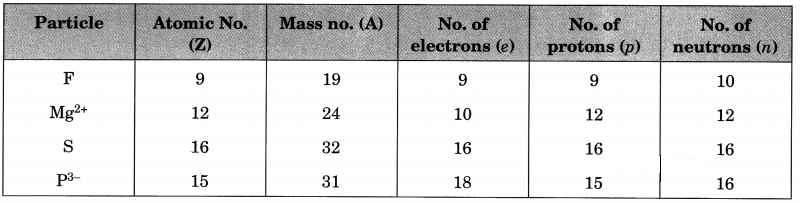Note: The value of positive charge on cation shows that the number of electrons in it are less than the number of protons by the same value. Similarly, the value of negative charge on anion shows that the number of electrons in it are more than the number of protons by the same value.